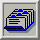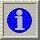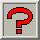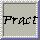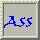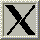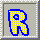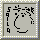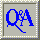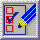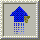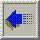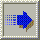##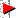3.18. Measuring Quantisation Error

From Heckbert (1982)

To measure the differences between the original and quantised images (the total quantisation error) we can use the following formula: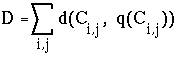where d(x,y) is the distance between two colours as previously looked at. Note that, since we are only comparing distances, we would not look at the actual distance but the distance squared, as square root operations are SLOW.

We define the "optimal" quantiser (for a given image and number of colours K) as the one which minimises D.